﻿ TT-01型 | 三木普利

#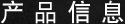## TT(01)型﻿TT(01)型

【规格】

TT-1X-011X0.20.51.01.52.02.53.03.54.00.20.518000.06×10-30.3
TT-2-01212345678100.20.518000.26×10-30.7
TT-2X-012X235810121518200.20.518000.52×10-31.0
TT-3-01358101520253035400.20.518001.23×10-31.5
TT-3X-013X1016203040506070800.20.518001.94×10-32.7
TT-4X-014X203050801001201501802000.20.550014.8×10-36.3

※上表设定的转矩值为转速1500min-1时的数值。

※设定转矩值有±20% 左右的偏差。

※＊部分的转矩需要耐久性时，请增大尺寸。

【尺寸】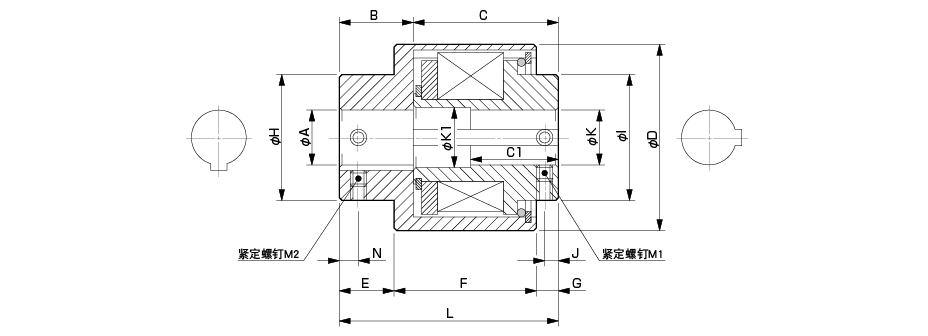1X12.52030234215305252235062-M42-M4
216.524.541.532.555203511353256672-M52-M5
2X20.531453465254011403857682-M52-M5
325.537.553.540753050114545591102-M62-M6
3X25.53685417530801145456121102-M62-M6
4X28.546956012035901680808141122-M102-M10

※尺寸4X 的φ K 在30mm 以上时，形状变直，无K1、C1 尺寸。

【转矩系数】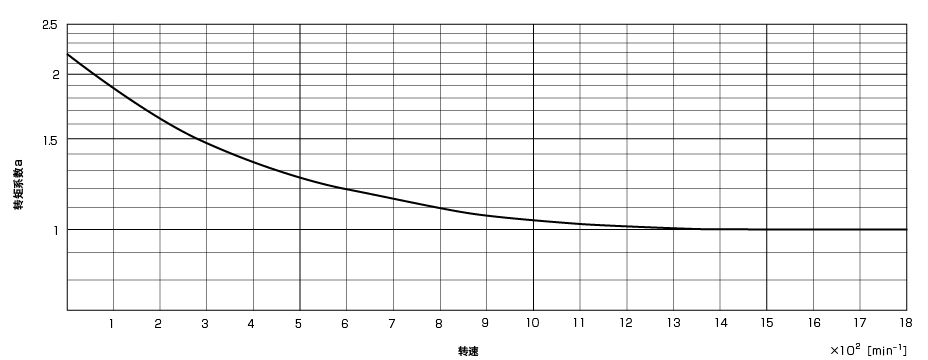【标准孔径φ A】

81011　12　1415　16　18192022242528303235384042454850
TT-1X-01符合JIS 旧标准 E9无标记

TT-2-01符合JIS 旧标准 E9无标记

TT-2X-01符合JIS 旧标准 E9无标记

TT-3-01符合JIS 旧标准 E9无标记

TT-3X-01符合JIS 旧标准 E9无标记

TT-4X-01符合JIS 旧标准 E9无标记

※孔径为φ 8mm 时，没有键槽。

【标准孔径φ K】

81011 12 1415 16 18192022242528303235384042454850
TT-1X-01符合JIS 旧标准 E9无标记

TT-2-01符合JIS 旧标准 E9无标记

TT-2X-01符合JIS 旧标准 E9无标记

TT-3-01符合JIS 旧标准 E9无标记

TT-3X-01符合JIS 旧标准 E9无标记

TT-4X-01符合JIS 旧标准 E9无标记

※孔径为φ 8mm 时，没有键槽。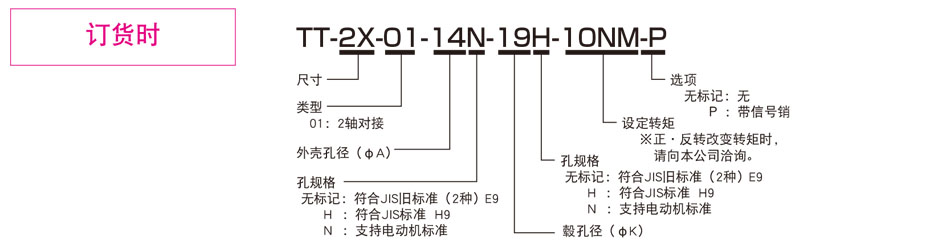## 信号销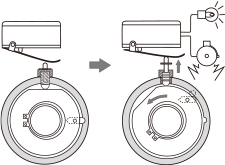【尺寸】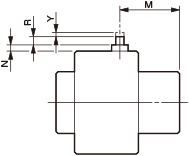1X 24 1.5 6.5 5.5
2 29 2.5 5 4.5
2X 36 2.5 5 4.5
3 43 2.5 5 4.5
3X 42 2.5 5 4.5
4X 55 2.5 5 2

【标准孔加工规格】

・紧定螺钉为产品附带。
・有关标明以外的孔加工标准尺寸，请向本公司洽询。
（根据毂尺寸可能无法加工。）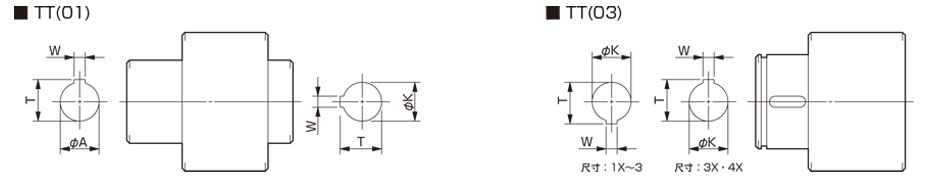（φA・φK）

(W)

(T)

（φA・φK）

(W)

(T)

（φA・φK）

(W)

(T)

H7

E9
- 公差
H7

H9
- 公差
G7
- -
8 8+0.0150 - - - - - - - - - -
10 10+0.0150 4+0.050+0.020 11.5+0.50 10Ｈ 10+0.0150 4+0.0300 11.8+0.50 - - - -
11 11+0.0180 4+0.050+0.020 12.5+0.50 11Ｈ 11+0.0180 4+0.0300 12.8+0.50 - - - -
12 12+0.0180 4+0.050+0.020 13.5+0.50 12Ｈ 12+0.0180 4+0.0300 13.8+0.50 - - - -
14 14+0.0180 5+0.050+0.020 16.0+0.50 14Ｈ 14+0.0180 5+0.0300 16.3+0.50 14Ｎ 14+0.024+0.006 5+0.0300 16.0+0.50
15 15+0.0180 5+0.050+0.020 17.0+0.50 15Ｈ 15+0.0180 5+0.0300 17.3+0.50 - - - -
16 16+0.0180 5+0.050+0.020 18.0+0.50 16Ｈ 16+0.0180 5+0.0300 18.3+0.50 - - - -
18 18+0.0180 5+0.050+0.020 20.0+0.50 18Ｈ 18+0.0180 6+0.0300 20.8+0.50 - - - -
19 19+0.0210 5+0.050+0.020 21.0+0.50 19Ｈ 19+0.0210 6+0.0300 21.8+0.50 19Ｎ 19+0.028+0.007 6+0.0300 21.5+0.50
20 20+0.0210 5+0.050+0.020 22.0+0.50 20Ｈ 20+0.0210 6+0.0300 22.8+0.50 - - - -
22 22+0.0210 7+0.061+0.025 25.0+0.50 22Ｈ 22+0.0210 6+0.0300 24.8+0.50 - - - -
24 24+0.0210 7+0.061+0.025 27.0+0.50 24Ｈ 24+0.0210 8+0.0360 27.3+0.50 24Ｎ 24+0.028+0.007 8+0.0360 27.0+0.50
25 25+0.0210 7+0.061+0.025 28.0+0.50 25Ｈ 25+0.0210 8+0.0360 28.3+0.50 - - - -
28 28+0.0210 7+0.061+0.025 31.0+0.50 28Ｈ 28+0.0210 8+0.0360 31.3+0.50 28Ｎ 28+0.028+0.007 8+0.0360 31.0+0.50
30 30+0.0210 7+0.061+0.025 33.0+0.50 30Ｈ 30+0.0210 8+0.0360 33.3+0.50 - - - -
32 32+0.0250 10+0.061+0.025 35.5+0.50 32Ｈ 32+0.0250 10+0.0360 35.3+0.50 - - - -
35 35+0.0250 10+0.061+0.025 38.5+0.50 35Ｈ 35+0.0250 10+0.0360 38.3+0.50 - - - -
38 38+0.0250 10+0.061+0.025 41.5+0.50 38Ｈ 38+0.0250 10+0.0360 41.3+0.50 38Ｎ 38+0.034+0.009 10+0.0360 41.0+0.50
40 40+0.0250 10+0.061+0.025 43.5+0.50 40Ｈ 40+0.0250 12+0.0430 43.3+0.50 - - - -
42 42+0.0250 10+0.061+0.025 45.5+0.50 42Ｈ 42+0.0250 12+0.0430 45.3+0.50 42Ｎ 42+0.034+0.009 12+0.0430 45.0+0.50
45 45+0.0250 12+0.075+0.032 48.5+0.50 45Ｈ 45+0.0250 14+0.0430 48.8+0.50 - - - -
48 48+0.0250 12+0.075+0.032 51.5+0.50 48Ｈ 48+0.0250 14+0.0430 51.8+0.50 48Ｎ 48+0.034+0.009 14+0.0430 51.5+0.50
50 50+0.0250 12+0.075+0.032 53.5+0.50 50Ｈ 50+0.0250 14+0.0430 53.8+0.50 - - - -
﻿